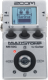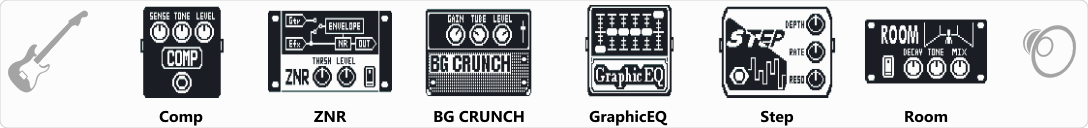# 29

Discussion in 'Zoom MS-50G' started by GILBERTO, Apr 11, 2020.

1. 29Device: Zoom Ms-50g
Firmware: 3.10

Optimized for: Guitar Amp

Effects chain:Effect: "Comp" (Dynamics / Filter), active - "yes"
"Sense" = 6
"Tone" = 6
"Level" = 80
"ATTCK" = Slow

Effect: "ZNR" (Dynamics / Filter), active - "yes"
"THRSH" = 10
"DETCT" = EfxIn
"Level" = 100

Effect: "BG CRUNCH" (Amp simulator), active - "yes"
"Gain" = 92
"Tube" = 30
"Level" = 70
"Trebl" = 50
"Middl" = 50
"Bass" = 50
"Prese" = 60
"CAB" = BG CRUNCH 1x12
"OUT" = LINE

Effect: "GraphicEQ" (Dynamics / Filter), active - "yes"
"160Hz" = 4
"400Hz" = 2
"800Hz" = 0
"3.2kHz" = 4
"6.4kHz" = 2
"12kHz" = 0
"Level" = 60

Effect: "Step" (Dynamics / Filter), active - "yes"
"Depth" = 62
"Rate" = 32
"Reso" = 5
"Shape" = 10
"Level" = 100

Effect: "Room" (Delay / Reverb), active - "yes"
"Decay" = 24
"Tone" = 6
"Mix" = 40
"PreD" = 5
"Level" = 100
"Tail" = Off

Note: This is a patch file, you will need to download and install the ToneLib-Zoom software to use the patch.

File size:
420 bytes
Views:
130